# 经典论文复现 | LSGAN：最小二乘生成对抗网络

2018/10/10 19:00

下载安装命令

## CPU版本安装命令

## GPU版本安装命令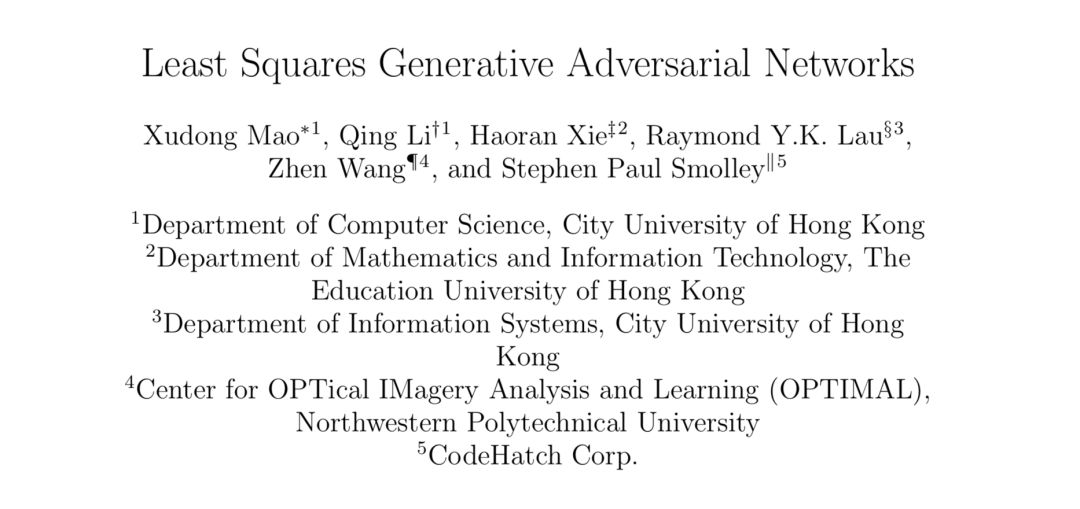LSGANs 这篇经典的论文主要工作是把交叉熵损失函数换做了最小二乘损失函数，这样做作者认为改善了传统 GAN 的两个问题，即传统 GAN 生成的图片质量不高，而且训练过程十分不稳定。

LSGANs 试图使用不同的距离度量来构建一个更加稳定而且收敛更快的，生成质量高的对抗网络。但是我看过 WGAN 的论文之后分析这一损失函数，其实并不符合 WGAN 作者的分析。在下面我会详细分析一下为什么 LSGANs 其实并没有那么好用。

http://aistudio.baidu.com/aistudio/#/projectdetail/25767

# LSGANs的优点

KL 散度就是 D(p||q) = H(p,q) - H(p)，它表示的是两个分布的差异，因为真实分布 p 的信息熵固定，所以一般由交叉熵来决定，所以这就是为什么传统 GAN 会采用交叉熵的缘故，论文也证明了 GAN 损失函数与 KL 散度的关系。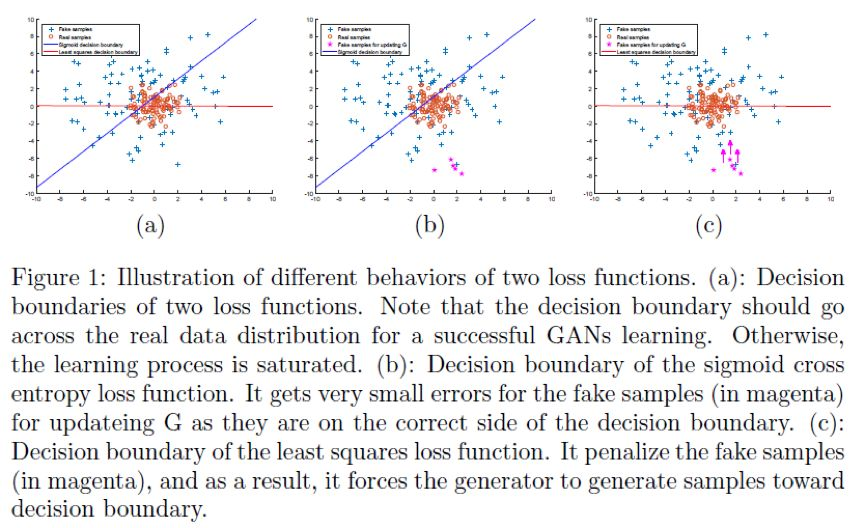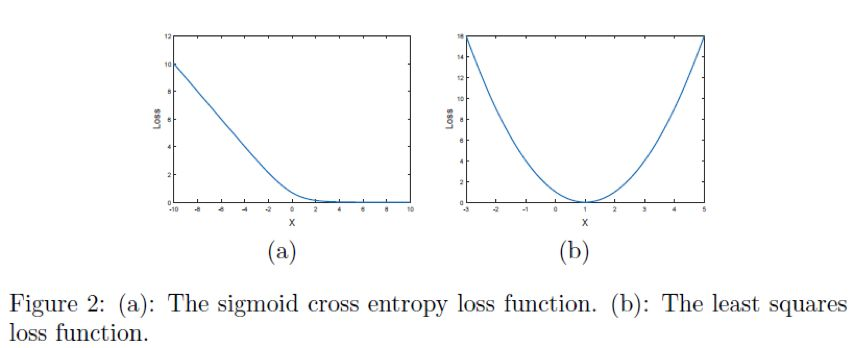# LSGANs的损失函数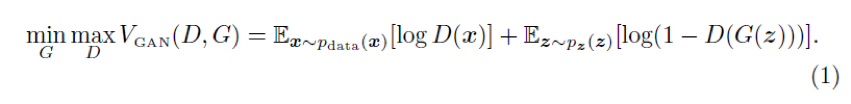LSGANs 的损失函数：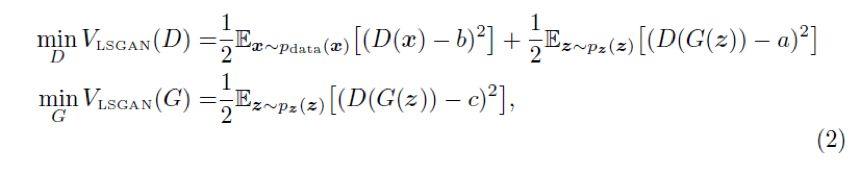def generator(z, name="G"):    with fluid.unique_name.guard(name+'_'):        fc1 = fluid.layers.fc(input = z, size = 1024)        fc1 = fluid.layers.fc(fc1, size = 128 * 7 * 7)        fc1 = fluid.layers.batch_norm(fc1,act = 'tanh')        fc1 = fluid.layers.reshape(fc1, shape=(-1, 128, 7, 7))        conv1 = fluid.layers.conv2d(fc1, num_filters = 4*64,                                    filter_size=5, stride=1,                                     padding=2, act='tanh')        conv1 = fluid.layers.reshape(conv1, shape=(-1,64,14,14))        conv2 = fluid.layers.conv2d(conv1, num_filters = 4*32,                                     filter_size=5, stride=1,                                    padding=2, act='tanh')        conv2 = fluid.layers.reshape(conv2, shape=(-1,32,28,28))        conv3 = fluid.layers.conv2d(conv2, num_filters = 1,                                     filter_size=5, stride=1,                                    padding=2,act='tanh')#         conv3 = fluid.layers.reshape(conv3, shape=(-1,1,28,28))        print("conv3",conv3)        return conv3
with fluid.unique_name.guard(name+'_'):
fc1 = fluid.layers.fc(input = z, size = 1024)
fc1 = fluid.layers.fc(fc1, size = 128 * 7 * 7)
fc1 = fluid.layers.batch_norm(fc1,act = 'tanh')
fc1 = fluid.layers.reshape(fc1, shape=(-1, 128, 7, 7))

conv1 = fluid.layers.conv2d(fc1, num_filters = 4*64,
filter_size=5, stride=1,
conv1 = fluid.layers.reshape(conv1, shape=(-1,64,14,14))

conv2 = fluid.layers.conv2d(conv1, num_filters = 4*32,
filter_size=5, stride=1,
conv2 = fluid.layers.reshape(conv2, shape=(-1,32,28,28))

conv3 = fluid.layers.conv2d(conv2, num_filters = 1,
filter_size=5, stride=1,
#         conv3 = fluid.layers.reshape(conv3, shape=(-1,1,28,28))
print("conv3",conv3)
return conv3


生成器代码展示

def discriminator(image, name="D"):    with fluid.unique_name.guard(name+'_'):        conv1 = fluid.layers.conv2d(input=image, num_filters=32,                                    filter_size=6, stride=2,                                    padding=2)        conv1_act = fluid.layers.leaky_relu(conv1)        conv2 = fluid.layers.conv2d(conv1_act, num_filters=64,                                     filter_size=6, stride=2,                                    padding=2)        conv2 = fluid.layers.batch_norm(conv2)        conv2_act = fluid.layers.leaky_relu(conv2)        fc1 = fluid.layers.reshape(conv2_act, shape=(-1,64*7*7))        fc1 = fluid.layers.fc(fc1, size=512)        fc1_bn = fluid.layers.batch_norm(fc1)        fc1_act = fluid.layers.leaky_relu(fc1_bn)        fc2 = fluid.layers.fc(fc1_act, size=1)        print("fc2",fc2)        return fc2
with fluid.unique_name.guard(name+'_'):
conv1 = fluid.layers.conv2d(input=image, num_filters=32,
filter_size=6, stride=2,
conv1_act = fluid.layers.leaky_relu(conv1)

conv2 = fluid.layers.conv2d(conv1_act, num_filters=64,
filter_size=6, stride=2,
conv2 = fluid.layers.batch_norm(conv2)
conv2_act = fluid.layers.leaky_relu(conv2)

fc1 = fluid.layers.reshape(conv2_act, shape=(-1,64*7*7))
fc1 = fluid.layers.fc(fc1, size=512)
fc1_bn = fluid.layers.batch_norm(fc1)
fc1_act = fluid.layers.leaky_relu(fc1_bn)

fc2 = fluid.layers.fc(fc1_act, size=1)
print("fc2",fc2)
return fc2


判别器代码展示

1. 使 b - c = 1，b - a = 2，例如 a = -1，b = 1，c = 0：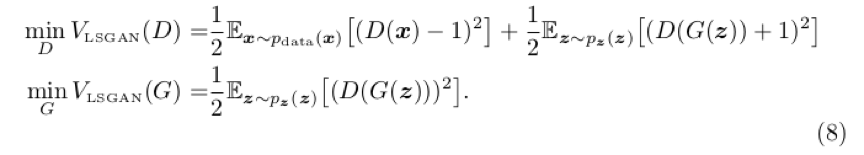2. 使 c = b，用 0-1 二元标签，我们可以得到：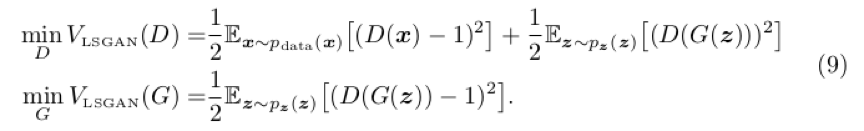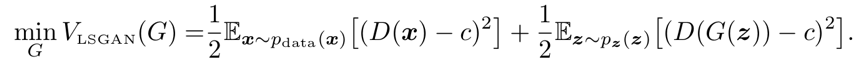# LSGANs未能解决的地方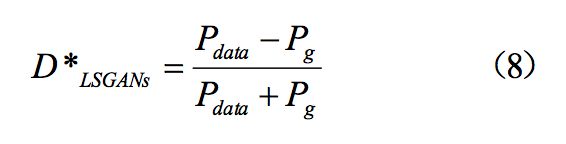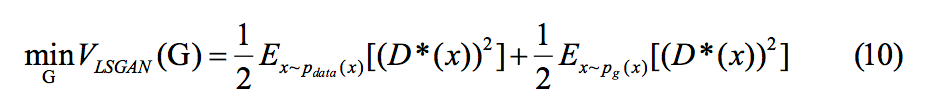1. P1(x)=0,P2(x)=0

2. P1(x)!=0,P2(x)!=0

3. P1(x)=0,P2(x)!=0

4. P1(x)!=0,P2(x)=0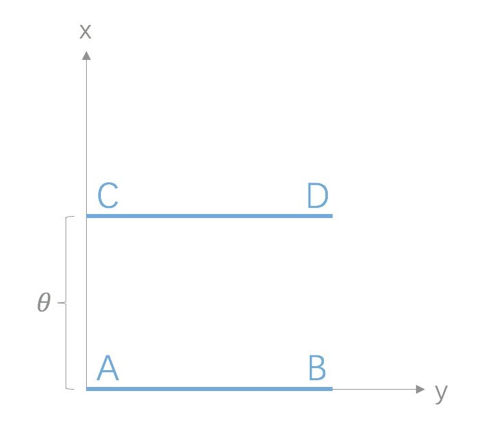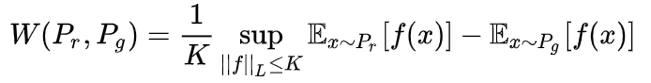# 两种模型架构和训练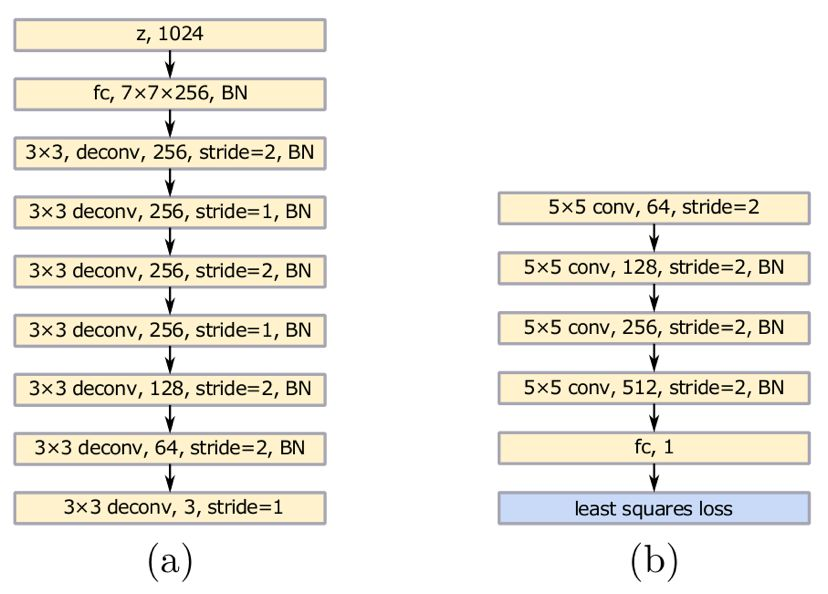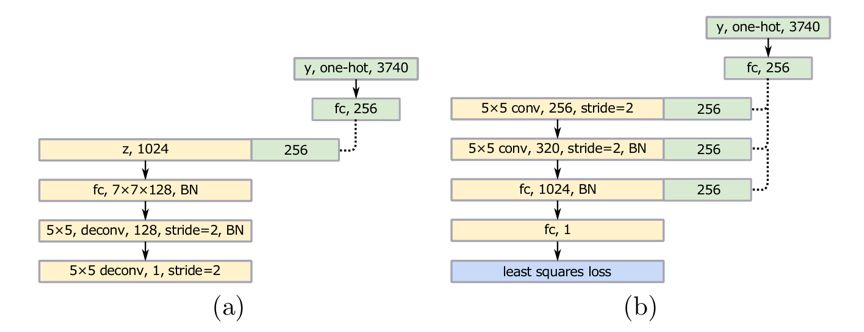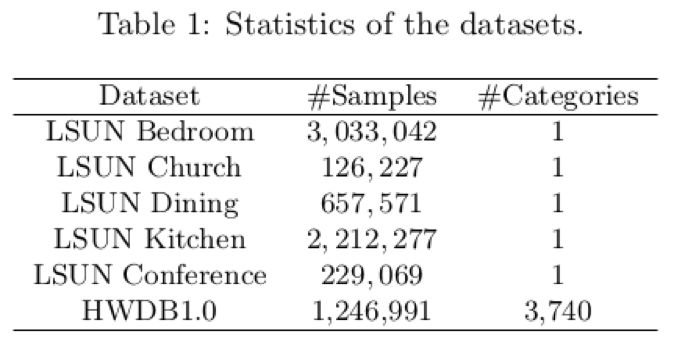本文使用的数据集列表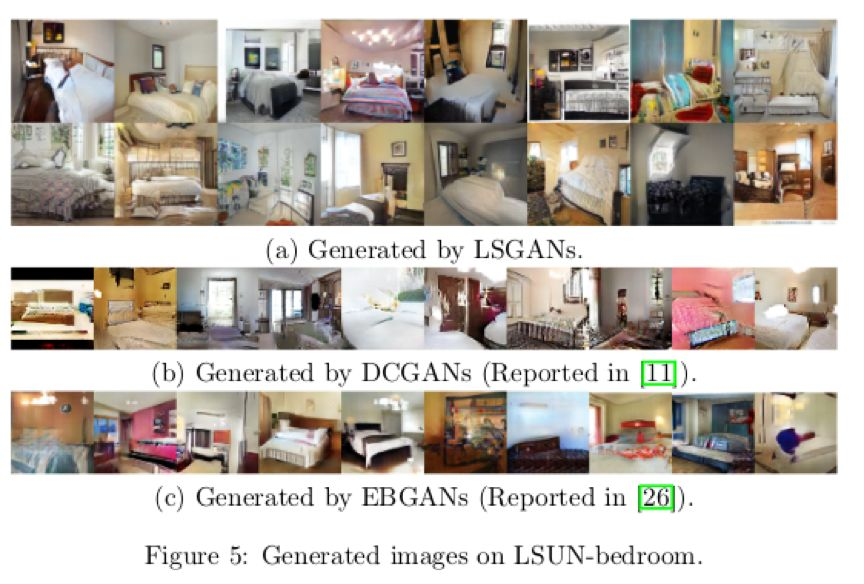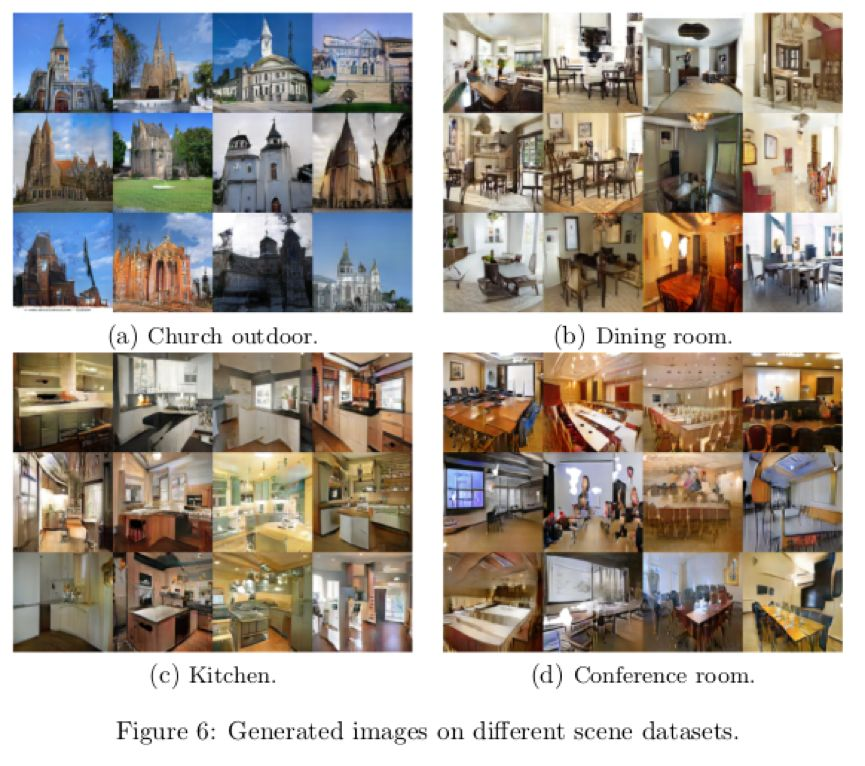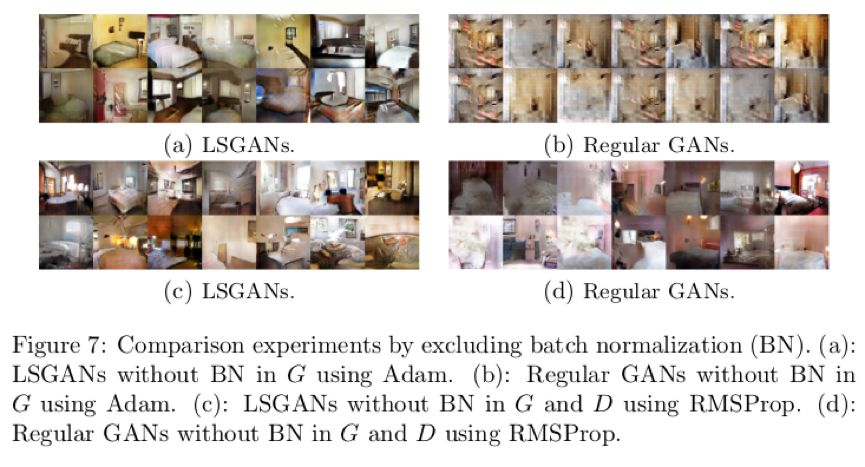1. 生成器 G 带有 batch normalization 批处理标准化（以下简称 BN）并且使用 Adam 优化器的话，LSGANs 生成的图片质量好，但是传统 GANs 从来没有成功学习到，会出现 mode collapse 现象；

2. 生成器 G 和判别器 D 都带有 BN 层，并且使用 RMSProp 优化器处理，LSGANs 会生成质量比 GANs 高的图片，并且 GANs 会出现轻微的 mode collapse 现象；

3. 生成器 G 带有 BN 层并且使用 RMSProp 优化器，生成器 G 判别器 D 都带有 BN 层并且使用 Adam 优化器时，LSGANs 与传统 GANs 有着相似的表现；

4. RMSProp 的表现比 Adam 要稳定，因为传统 GANs 在 G 带有 BN 层时，使用 RMSProp 优化可以成功学习，但是使用 Adam 优化却不行。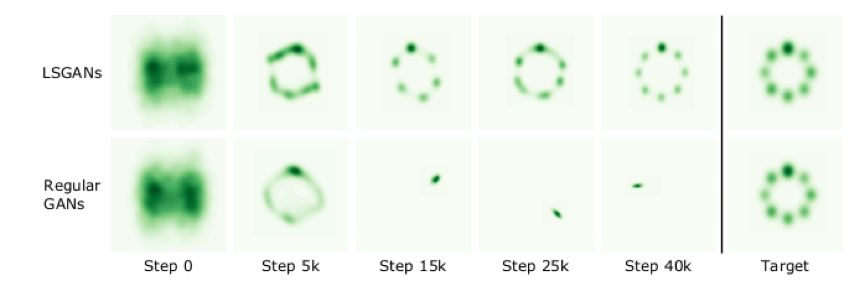# 论文具体实现

LSGANs：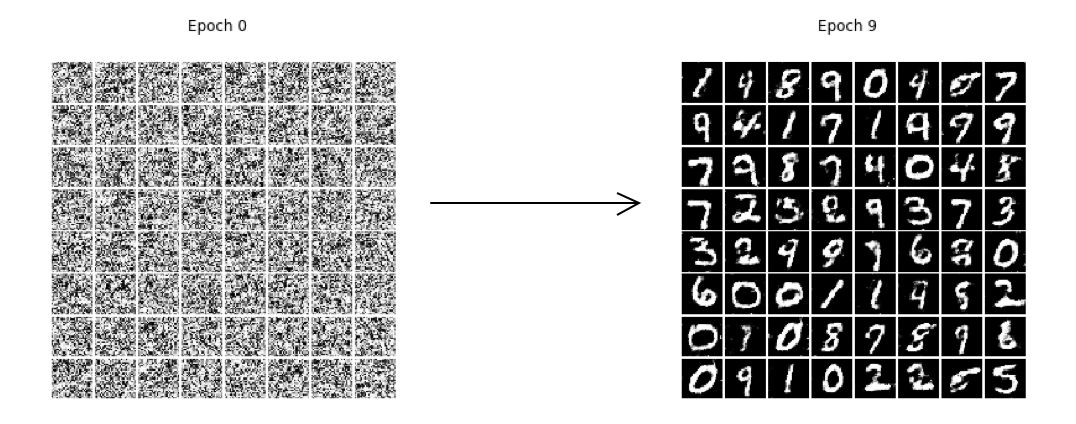GAN：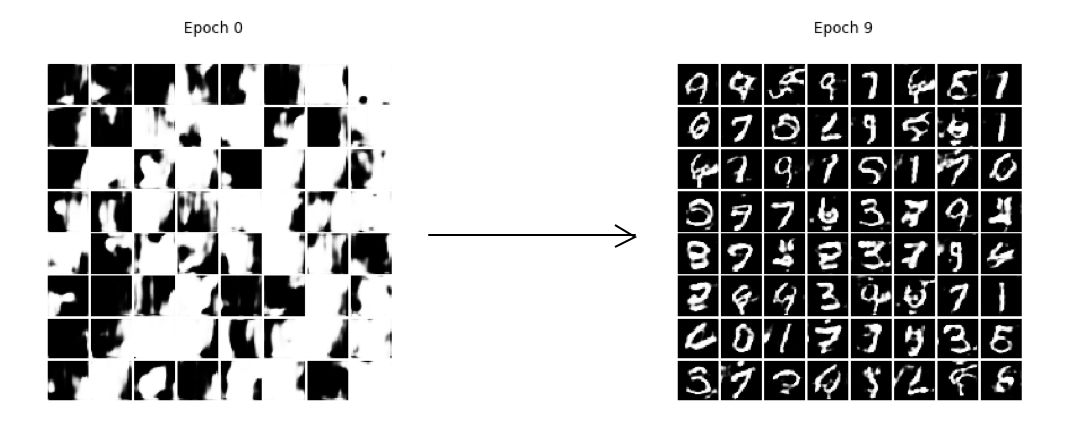# 总结

LSGANs 是对 GAN 的一次优化，从实验的情况中，笔者也发现了一些奇怪的现象。我本来是参考论文把判别器 D 的损失值，按真假两种 loss 加起来一并放入 Adam 中优化，但是无论如何都学习不成功，梯度还是弥散了，最后把 D_fake_loss 和 D_real_loss 分为两个 program，放入不同的 Adam 中优化判别器D 的参数才达到预期效果。

下载安装命令

## CPU版本安装命令

## GPU版本安装命令


PaddlePaddle团队：关于如何使用 GPU 运行，可以看下执行器 Executor（单 GPU 或单线程 CPU 执行器）或 ParallelExecutor（多 GPU 或多线程 CPU 执行器，也可以单 GPU/线程 CPU 执行）的文档，前者指定 place 为 CUDAPlace，后者接口有个 use_cuda，具体请参考文档。也可以看 models repo 例子，比如 image_classification 或 text_classification 的例子。

PaddlePaddle团队：关于 Program 设计可以参考官方文档。这里提一点，在用户使用的直观感受中和 TensorFlow graph 不同的是，凡是放在一个 Program 里 op，只要运行该 Program，这些 op 就都会执行；而 TensorFlow，指定一个 variable，只运行以该 variable 为叶子节点的 graph，其他多余 node 不执行，这是最大的用户感受到的区别。

# 参考文献

. I. Goodfellow, J. Pouget-Abadie, M. Mirza, B. Xu, D. Warde-Farley, S. Ozair, A. Courville, and Y. Bengio, “Generative adversarial nets,” in Advances in Neural Information Processing Systems (NIPS), pp. 2672–2680, 2014.

. M. Arjovsky, S. Chintala, and L. Bottou. Wasserstein GAN. arXiv preprint arXiv:1701.07875, 2017.

. Andrew Brock, Jeff Donahue and Karen Simonyan. Large Scale GAN Training for High Fidelity Natural Image Synthesis. arXiv:1809.11096, 2018.

. Schlegl, Thomas, et al. "Unsupervised Anomaly Detection with Generative Adversarial Networks to Guide Marker Discovery." arXiv preprint arXiv:1703.05921 (2017).

. https://zhuanlan.zhihu.com/p/25071913?from_voters_page=true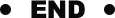### 作者的其它热门文章

0
0 收藏

0 评论
0 收藏
0